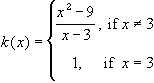#Interactive Real Analysis

Next | Previous | Glossary | Map

## 6.3. Discontinuous Functions

### Examples 6.3.4(a):

Prove that k(x) has a removable discontinuity at x = 3, and draw the graph of k(x).We can easily check (by factoring the numerator) that the limit as x approaches 3 from the right and from the left is equal to 6. Hence, the limit as x approaches 3 exists, and therefore the function has a removable discontinuity at x = 3. If we define k(3) = 6 instead of k(3) = 1 then the function, in fact, will be continuous on the real line.

Next | Previous | Glossary | Map